Learning Library
Who we are
Guidance

# Time for Times Tables! 10 Printables

Times tables can be tricky. Help fourth graders master multiplication with these math worksheets.

Click on a worksheet in the set below to see more info or download the PDF.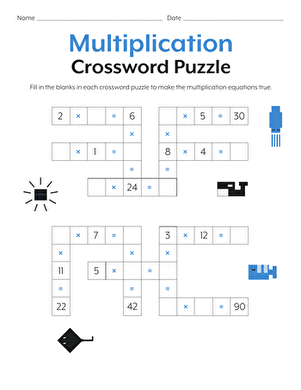### Multiplication Crossword

Have some fun reviewing times tables with this multiplication crossword.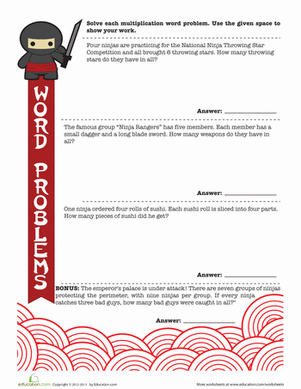### Ninja Word Problems

Learn the way of the math ninja with multiplication word problems! Your master mathematician will review times tables as she works through each word problem.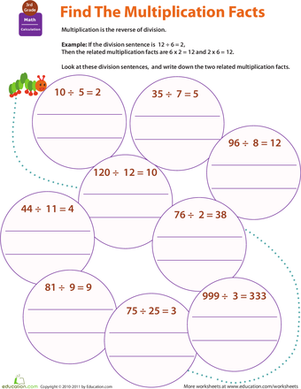### Multiplication Facts

Give your fourth grader practice with an important concept: fact families. This one explores the multiplication facts that relate to division facts.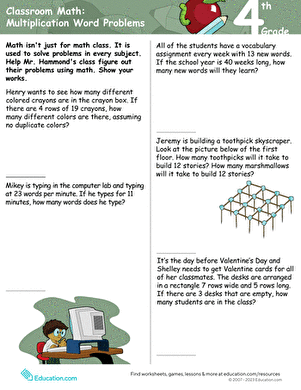### Classroom Math: Multiplication Word Problems

Your fourth grader will be challenged to complete five word problems using multiplication with two-digit numbers.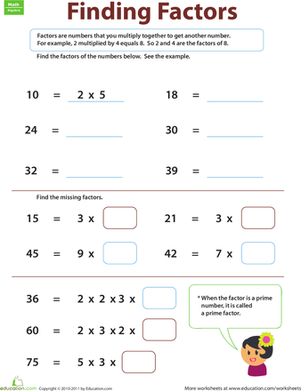### Finding Factors

With this worksheet, your child will be learning about factors, and she'll be getting multiplication practice as she goes.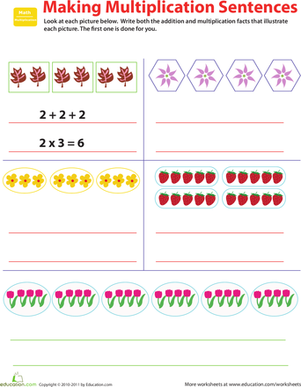### Related Facts: Make Multiplication Sentences

Who knew that multiplication and addition were so closely related? Give your fourth grader practice with the concept, with this valuable worksheet.

The set is continued below.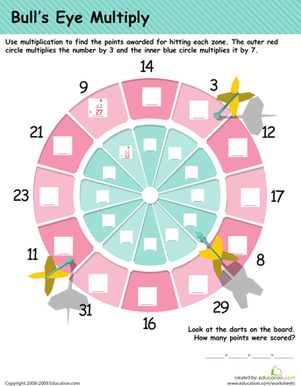### Multiplication Practice: Darts!

In this 4th grade math worksheet, your child will practice his multiplication tables as he calculates the points on a dart board.### 2-Digit Multiplication

Challenge your fourth grader with 2-digit multiplication. Give him a hand practicing multiplying double digits by double digits with this ninja worksheet.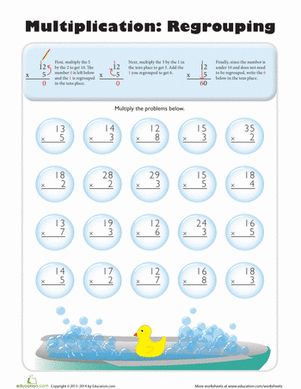### Multiplication Regrouping

This worksheet is jam-packed with 2 pages of multiplication regrouping problems to help kids tackle two-digit multiplication.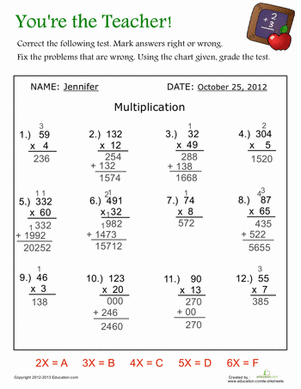### You're the Teacher!

No reason to claim the dog ate your homework or an alien abducted your notes -- for this math assignment, you'll be the grader!